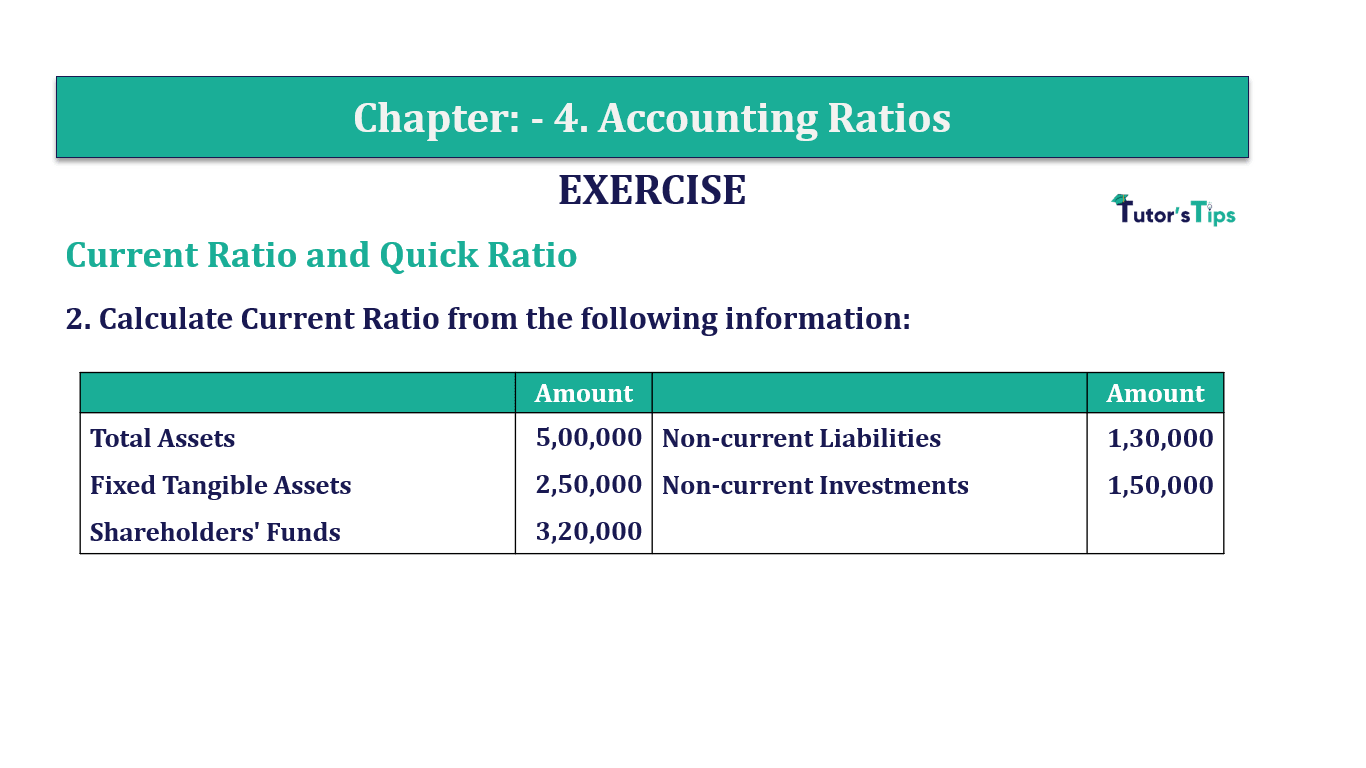# Question 2 Chapter 4 of +2-B – T.S. Grewal 12 ClassQuestion No. 2 - Chapter No.4 - T.S. Grewal +2 Book Part B

Question 2 Chapter 4 of +2-B

Current Ratio and Quick Ratio

2. Calculate Current Ratio from the following information:

 Amount Amount Total Assets 5,00,000 Non-current Liabilities 1,30,000 Fixed Tangible Assets 2,50,000 Non-current Investments 1,50,000 Shareholders’ Funds 3,20,000

### The solution of Question 2 Chapter 4 of +2-B: –

 Total Assets Fixed Tangible Assets + Non-Current Investments + Current Assets

 Rs.5,00,000 = Rs.2,50,000 + Rs.1,50,000 + Current Assets

 Current Assets =Rs.5,00,000 – Rs.4,00,000 = Rs. 1,00,000

 Total Liabilities = Shareholder’s Funds + Non – Current Liabilities + CurrentLiabilities

 Rs.5,00,000 = Rs.3,20,000 + Rs.1,30,000 + Current Liabilities

 Current Liabilities = Rs.5,00,000 – Rs.4,50,000 = Rs. 50,000

 Current Ratio= Current Assets Current Liabilities

 Current Ratio= 1,00,000 50,000

Current Ratio=2:1

Balance Sheet: Meaning, Format & Examples

Comment if you have any question.

Also, Check out the solved question of previous Chapters: –